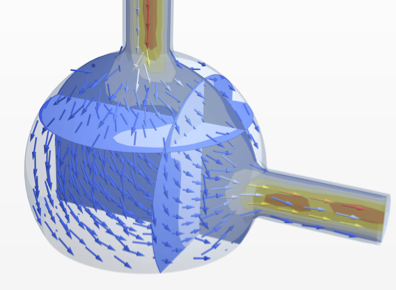# Nonlinear Finite Elements

Version 12 extends its numerical partial differential equation-solving capabilities to solve nonlinear partial differential equations over arbitrary-shaped regions with the finite element method. Given a nonlinear, possibly coupled partial differential equation (PDE), a region specification and boundary conditions, the numerical PDE-solving capabilities find solutions to stationary and time-dependent nonlinear partial differential equations. This functionality will enable you to model a much larger class of industry-relevant applications from domains like physics, chemistry, mechanics, fluid dynamics and many more.• Support for stationary nonlinear PDEs over regions. »
• Model with PDEs depending on space, time and the dependent. »
• Use PDEs depending on the derivative of the dependent. »
• Solve complex-valued nonlinear PDEs. »
• Specify nonlinear generalized Neumann boundary conditions. »
• Solve time-dependent nonlinear PDEs over regions. »
• Transient problems use automatic time-stepping techniques.
• Formulate coupled nonlinear PDEs for multi-physics analysis. »
• Solve computational fluid dynamics problems. »
• Program with the finite element method interface.
• Use a Newton algorithm for root finding of large systems of equations. »